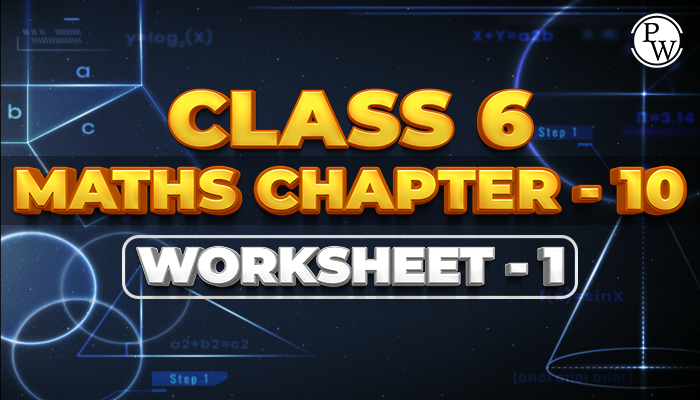# Class 6 Maths Chapter 10 Worksheet - 1

## Mensuration

Mensuration is a branch of mathematics that mainly deals with the study of different kinds of Geometrical shapes along with their area, length, volume, and perimeters. It's completely based on the application of both algebraic equations and geometric calculations. The results which are obtained by the Mensuration are considered to be very accurate.### Solved Questions

Q1. Find the perimeter of a rectangle whose length and breadth are 25 cm and 15 cm respectively.

1. 100 cm
2. 120 cm
3. 80 cm
4. 40 cm

Ans. The correct option is 3

Length of rectangle = 25cm Breadth of rectangle = 15cm

Perimeter = 2 (l + b) = 2(25+15) = 2 × 40 = 80cm.

Q2. Find the perimeter of a square whose side is 5 cm

1. 5 cm
2. 10 cm
3. 15 cm
4. 20 cm

Ans. The correct option is 4

Side of square = 5cm

Perimeter of square = 4 × side, perimeter = 4 × 5 = 20cm

Q3. Find the side of a square whose perimeter is 100 cm

1. 400 cm
2. 25 cm
3. 200 cm
4. 50 cm

Ans. The correct option is 2

Perimeter of square = 100cm

Side = Perimeter/4 =  100/4 = 25cm

Q4. Find the cost of fencing a square park of side 300m at the rate of Rs 20 per metre.

1. Rs 24000
2. Rs 20000
3. Rs 16000
4. Rs 28000

Ans. The Correct option is 1

Side of square park = 300m

Perimeter = 4 x side = 4 x 300 = 1200m

Cost of fencing 1200 metre at the rate of Rs 20 per metre = 20 × 1200 = Rs 24000

Q5. Find the perimeter of an equilateral triangle of side 9cm.

1. 36cm
2. 8cm
3. 27cm
4. 45cm

Ans. The correct option is 3

Side of an equilateral triangle = 9 cm

All three sides of equilateral triangle are equal. So, perimeter = 3 × side = 3 × 9 = 27cm.

Q6. Find the area of a rectangle whose length is 4cm and breadth is 2cm.

1. 8 cm2
2. 12 cm2
3. 6 cm2
4. 10 cm2

Ans. The correct Option is 1 Length of rectangle = 4 cm Breadth of rectangle = 2cm

Area of rectangle = l × b = 4 × 2 = 8 cm2

Q7. Find the area of a rectangle if its length and breadth are 28cm and 120mm respectively.

1. 3360cm2
2. 3360mm2
3. 336cm2
4. 475cm2

Ans. The correct option is 1

Length of rectangle = 28cm Breadth of rectangle = 120mm

1cm = 10mm, 28cm = 28 × 10 = 280mm so, length = 280mm

area = l × b = 280 × 120 = 3360 mm2

Before solving the worksheet of Physics Wallah it is highly recommended to read the theory of the chapter and solve the questions given in your textbook. For reference, students can also check the CBSE Class 6 maths notes which are prepared by an expert team of Physics Wallah. Do visit NCERT Solutions for class 6 maths which has the best solutions among all other websites.

### Also Check